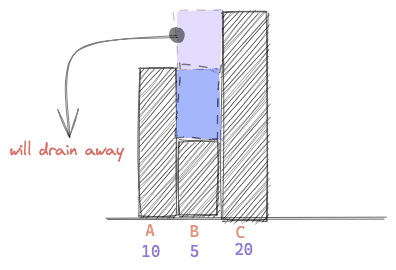# Trapping Rain Water 💦 (Leetcode 42)The Problem Given `n` non-negative integers representing an elevation map where the width of each bar is `1`, compute how much water it can trap after raining.

Example 1:

```Input: height = [0,1,0,2,1,0,1,3,2,1,2,1]
Output: 6
Explanation: The above elevation map (black section) is represented by array [0,1,0,2,1,0,1,3,2,1,2,1]. In this case, 6 units of rain water (blue section) are being trapped.
```

Example 2:

```Input: height = [4,2,0,3,2,5]
Output: 9
```

Constraints:

• `n == height.length`
• `1 <= n <= 2 * 104`
• `0 <= height[i] <= 105`

Source: Leetcode

If you have come to see the solution before trying to solve it yourself, this really won’t help you, promise! If that is the case, we will recommend, please go back and first try it yourself. If you can’t solve it after (at least)an hour of thinking and trying, please come back! 🙂 You can solve it here

__________________________________________________________

Solution

Consider we have only three elevations named A, B and C with heights [10, 5, 20]. If we continue to pour water on elevation 5, it will remain on top of 5 until it reaches 10. Anything above 10 will be drained away as show below.

So, for B, the effective height of water is the minimum height of all the maximum heights that belong to both sides of B.

In other words, for any elevation,

effective height for B = min ( max(all heights to the left of B), max(all heights to the right of B))

Before jumping into the code, let’s do a little simulation of the idea of effective height and trapped rain water calculation.

Consider we have 5 elevation points with heights [10, 0, 5, 0, 20] as below.

(Sorry for the drawing mistake, height of E doesn’t look proportionate to A or C 😛 That won’t hurt understanding the solution though )

Let’s start with elevation point A. There is no point left to it, so, the max height to A is the height of itself, 10. There are 4 points right to A with heights [0, 5, 0, 20]. The max is 20. So, the minimum between left max and right max is 10. So, the effective height is 10 – 10 = 0. That means, elevation point A cannot trap any water.

In the same way, max height to the left of B is 10 and to the right of B is 20. Effective height is min(10, 20) = 10 and B can trap 10 – 0 = 10 units of water.

Next three images show with calculation how much water C, D and E can trap.

So, the total unit of water trapped will be 0 + 10 + 5 + 10 + 0 = 25.

The Algorithm

Runtime complexity can easily be improved to O(N) if we apply simple Dynamic Programming and store the max value so far from the beginning of the array in another array called left. Also, we can store the same starting from the end of the array in another array called right. Then each time we want to know the max values of left and right of an elevation point, we will simply check the left and right array.

So, formally, the algorithm is:

1. Start from i = 0 to end and store max value in left[ ] array. Do Something like left [i] = max(left[i – 1], height [i] )
2. Start from i = end to 0 and store max value in left[ ] array. Do Something like right [i] = max(right[i + 1], height [i] )
3. Now, loop through the height array and take effective height as, effective_height = min (left[i], right[I]). Add trapped water unit.

Code

``````int trap(vector<int>& height) {
int N = height.size();

vector<int> left(N, 0);
vector<int> right(N, 0);

left = height;
right[N - 1] = height[N - 1];

for(int i = 1; i < N; i++){
left[i] = max(left[i - 1], height[i]);
right[N - i - 1] = max(height[N - i - 1], right[N - i]);
}

int ans = 0;

for(int i = 0; i < N; i++){
ans += min(left[i], right[i]) - height[i];
}

return ans;
}``````

Happy Coding !!! 😀